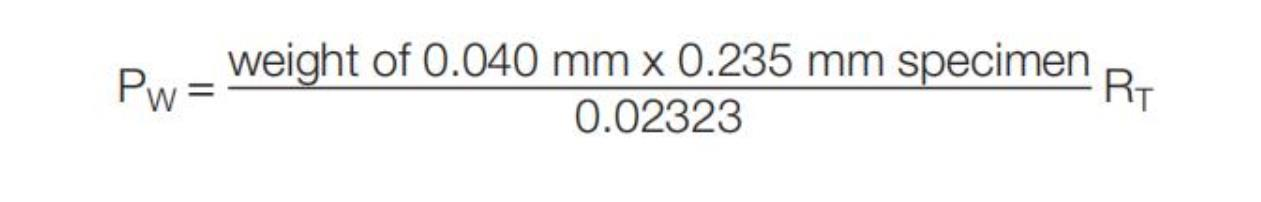1 Scope This test method is used to determine the resistivity of copper foil.

2 Applicable Documents ASTM-B-193 Resistivity of Conductive Materials.

3 Test Specimen

3.1 Three samples should be selected at equal distances across the width of the material from each lot and the width and gauge length measured to the nearest 0.025 mm.

4 Equipment/Apparatus

4.1 Tester The resistance of the samples shall be measured with instruments of suitable sensitivity (see ASTM-B-193).

5 Procedure

5.1 Test

5.1.1 Resistance Determination

Three samples shall be from each lot and the width and gauge length measured to the nearest 0.025 mm. The resistance of the samples shall be measured with instruments of suitable sensitivity, in accordance with ASTM-B-193.

5.1.2 For convenience, the distance between test points may be 15 cm, and the weight of the 2.5 cm wide sample being measured is determined by weighing a 2.5 cm x 15 cm strip from the test specimen.

5.2 Evaluation

5.2.1 Calculate the resistance using the formula:where:
T = reference temperature (20°C)
t = temperature at which measurement is made (°C)
αT = temperature coefficient of resistance (0.00388)
RT = resistance at reference temperature (20°C)
Rt = measured resistance

5.2.2
Calculate weight resistivity in ohms – gram/meter2 using the formula:where:
W = weight of test specimen (grams)
L1 = gauge length (meters)
L2 = length of test specimen (meters)
RT = resistance at reference temperature (20°C)
Note: If the procedure described in 5.1.2 is used, then:
L1 L2 Therefore is (0.1524 meters)2 or: